## Phase Transitions

### Learning Objectives

By the end of this section, you will be able to:

• Define phase transitions and phase transition temperatures
• Explain the relation between phase transition temperatures and intermolecular attractive forces
• Describe the processes represented by typical heating and cooling curves, and compute heat flows and enthalpy changes accompanying these processes

We witness and utilize changes of physical state, or phase transitions, in a great number of ways. As one example of global significance, consider the evaporation, condensation, freezing, and melting of water. These changes of state are essential aspects of our earth’s water cycle as well as many other natural phenomena and technological processes of central importance to our lives. In this module, the essential aspects of phase transitions are explored.

## Vaporization and Condensation

When a liquid vaporizes in a closed container, gas molecules cannot escape. As these gas phase molecules move randomly about, they will occasionally collide with the surface of the condensed phase, and in some cases, these collisions will result in the molecules re-entering the condensed phase. The change from the gas phase to the liquid is called condensation. When the rate of condensation becomes equal to the rate of vaporization, neither the amount of the liquid nor the amount of the vapor in the container changes. The vapor in the container is then said to be in equilibrium with the liquid. Keep in mind that this is not a static situation, as molecules are continually exchanged between the condensed and gaseous phases. Such is an example of a dynamic equilibrium, the status of a system in which reciprocal processes (for example, vaporization and condensation) occur at equal rates. The pressure exerted by the vapor in equilibrium with a liquid in a closed container at a given temperature is called the liquid’s vapor pressure (or equilibrium vapor pressure). The area of the surface of the liquid in contact with a vapor and the size of the vessel have no effect on the vapor pressure, although they do affect the time required for the equilibrium to be reached. We can measure the vapor pressure of a liquid by placing a sample in a closed container, like that illustrated in Figure 1, and using a manometer to measure the increase in pressure that is due to the vapor in equilibrium with the condensed phase.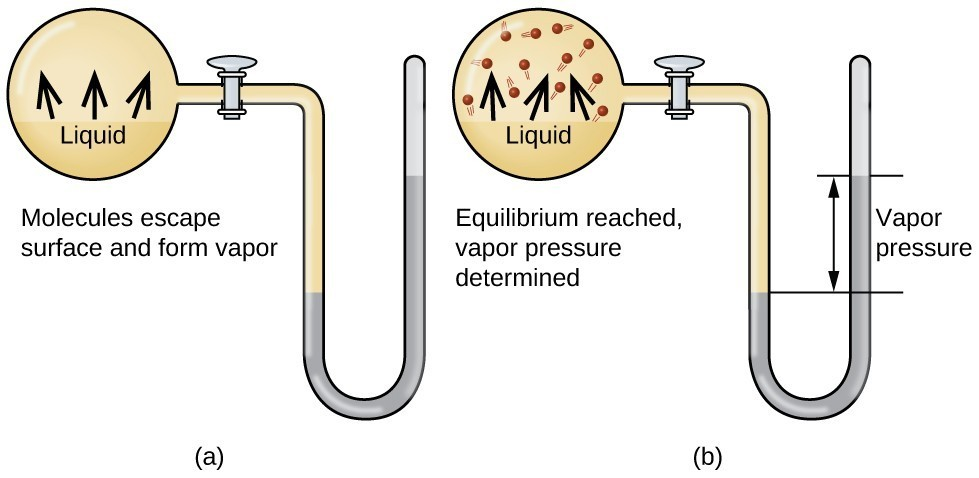Figure 1. In a closed container, dynamic equilibrium is reached when (a) the rate of molecules escaping from the liquid to become the gas (b) increases and eventually (c) equals the rate of gas molecules entering the liquid. When this equilibrium is reached, the vapor pressure of the gas is constant, although the vaporization and condensation processes continue.

The chemical identities of the molecules in a liquid determine the types (and strengths) of intermolecular attractions possible; consequently, different substances will exhibit different equilibrium vapor pressures. Relatively strong intermolecular attractive forces will serve to impede vaporization as well as favoring “recapture” of gas-phase molecules when they collide with the liquid surface, resulting in a relatively low vapor pressure. Weak intermolecular attractions present less of a barrier to vaporization, and a reduced likelihood of gas recapture, yielding relatively high vapor pressures. The following example illustrates this dependence of vapor pressure on intermolecular attractive forces.

### Example 1: Explaining Vapor Pressure in Terms of IMFs

Given the shown structural formulas for these four compounds, explain their relative vapor pressures in terms of types and extents of IMFs: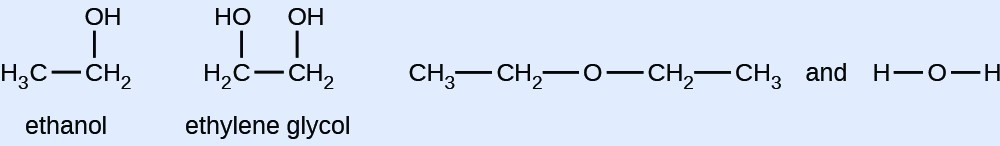At 20 °C, the vapor pressures of several alcohols are given in this table. Explain these vapor pressures in terms of types and extents of IMFs for these alcohols:

 Compound methanol CH3OH ethanol C2H5OH propanol C3H7OH butanol C4H9OH Vapor Pressure at 25 °C 11.9 kPa 5.95 kPa 2.67 kPa 0.56 kPa

As temperature increases, the vapor pressure of a liquid also increases due to the increased average KE of its molecules. Recall that at any given temperature, the molecules of a substance experience a range of kinetic energies, with a certain fraction of molecules having a sufficient energy to overcome IMF and escape the liquid (vaporize). At a higher temperature, a greater fraction of molecules have enough energy to escape from the liquid, as shown in Figure 2. The escape of more molecules per unit of time and the greater average speed of the molecules that escape both contribute to the higher vapor pressure.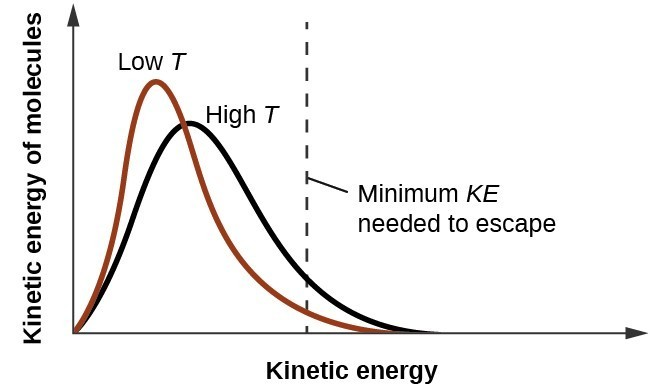Figure 2. Temperature affects the distribution of kinetic energies for the molecules in a liquid. At the higher temperature, more molecules have the necessary kinetic energy, KE, to escape from the liquid into the gas phase.

## Boiling Points

When the vapor pressure increases enough to equal the external atmospheric pressure, the liquid reaches its boiling point. The boiling point of a liquid is the temperature at which its equilibrium vapor pressure is equal to the pressure exerted on the liquid by its gaseous surroundings. For liquids in open containers, this pressure is that due to the earth’s atmosphere. The normal boiling point of a liquid is defined as its boiling point when surrounding pressure is equal to 1 atm (101.3 kPa). Figure 3 shows the variation in vapor pressure with temperature for several different substances. Considering the definition of boiling point, these curves may be seen as depicting the dependence of a liquid’s boiling point on surrounding pressure.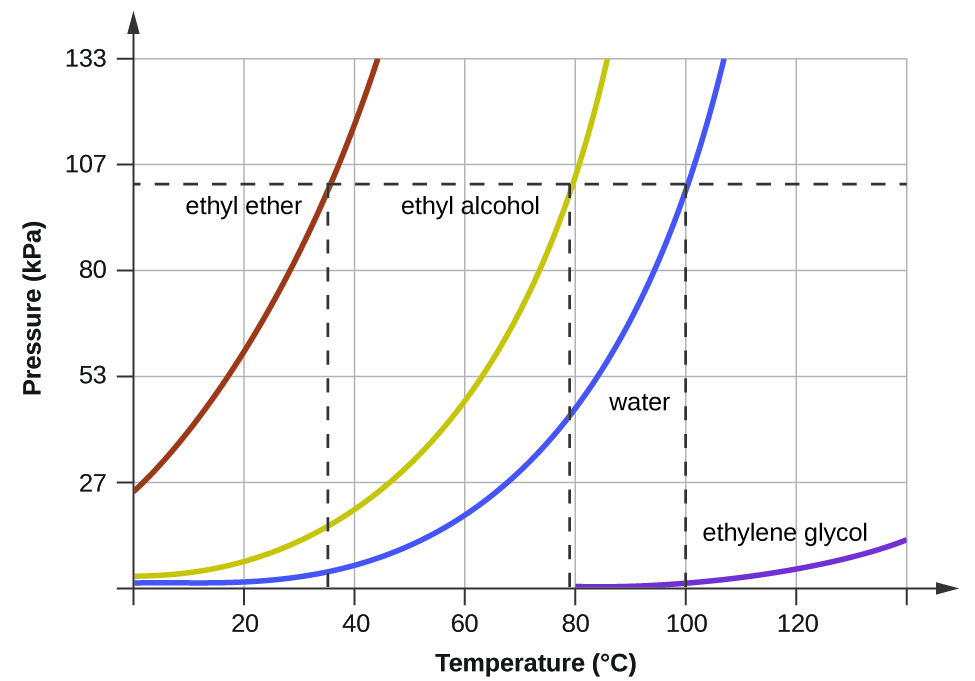Figure 3. The boiling points of liquids are the temperatures at which their equilibrium vapor pressures equal the pressure of the surrounding atmosphere. Normal boiling points are those corresponding to a pressure of 1 atm (101.3 kPa.)

### Example 2: A Boiling Point at Reduced Pressure

A typical atmospheric pressure in Leadville, Colorado (elevation 10,200 feet) is 68 kPa. Use the graph in Figure 3 to determine the boiling point of water at this elevation.

The boiling point of ethyl ether was measured to be 10 °C at a base camp on the slopes of Mount Everest. Use Figure 3 to determine the approximate atmospheric pressure at the camp.

The quantitative relation between a substance’s vapor pressure and its temperature is described by the Clausius-Clapeyron equation:

$P=A{e}^{-\Delta {H}_{\text{vap}}\text{/}RT}$

where ΔHvap is the enthalpy of vaporization for the liquid, R is the gas constant, and ln A is a constant whose value depends on the chemical identity of the substance. This equation is often rearranged into logarithmic form to yield the linear equation:

$\text{ln}P=-\frac{\Delta {H}_{\text{vap}}}{RT}+\text{ln}A$

This linear equation may be expressed in a two-point format that is convenient for use in various computations, as demonstrated in the example exercises that follow. If at temperature T1, the vapor pressure is P1, and at temperature T2, the vapor pressure is T2, the corresponding linear equations are:

$\text{ln}{P}_{1}=-\frac{\Delta {H}_{\text{vap}}}{R{T}_{1}}+\text{ln}A\text{and}\text{ln}{P}_{2}=-\frac{\Delta {H}_{\text{vap}}}{R{T}_{2}}+\text{ln}A$

Since the constant, ln A, is the same, these two equations may be rearranged to isolate ln A and then set them equal to one another:

$\text{ln}{P}_{1}+\frac{\Delta {H}_{\text{vap}}}{R{T}_{1}}=\text{ln}{P}_{2}+\frac{\Delta {H}_{\text{vap}}}{R{T}_{2}}$

which can be combined into:

$\text{ln}\left(\frac{{P}_{2}}{{P}_{1}}\right)=\frac{\Delta {H}_{\text{vap}}}{R}\left(\frac{1}{{T}_{1}}-\frac{1}{{T}_{2}}\right)$

### Example 3: Estimating Enthalpy of Vaporization

Isooctane (2,2,4-trimethylpentane) has an octane rating of 100. It is used as one of the standards for the octane-rating system for gasoline. At 34.0 °C, the vapor pressure of isooctane is 10.0 kPa, and at 98.8 °C, its vapor pressure is 100.0 kPa. Use this information to estimate the enthalpy of vaporization for isooctane.

At 20.0 °C, the vapor pressure of ethanol is 5.95 kPa, and at 63.5 °C, its vapor pressure is 53.3 kPa. Use this information to estimate the enthalpy of vaporization for ethanol.

### Example 4: Estimating Temperature (or Vapor Pressure)

For benzene (C6H6), the normal boiling point is 80.1 °C and the enthalpy of vaporization is 30.8 kJ/mol. What is the boiling point of benzene in Denver, where atmospheric pressure = 83.4 kPa?

For acetone (CH3)2CO, the normal boiling point is 56.5 °C and the enthalpy of vaporization is 31.3 kJ/mol. What is the vapor pressure of acetone at 25.0 °C?

## Enthalpy of Vaporization

Vaporization is an endothermic process. The cooling effect can be evident when you leave a swimming pool or a shower. When the water on your skin evaporates, it removes heat from your skin and causes you to feel cold. The energy change associated with the vaporization process is the enthalpy of vaporization, ΔHvap. For example, the vaporization of water at standard temperature is represented by:

${\text{H}}_{2}\text{O(}l\text{)}\longrightarrow {\text{H}}_{2}\text{O(}g\text{)}\Delta {H}_{\text{vap}}=\text{44.01 kJ/mol}$

As described in the chapter on thermochemistry, the reverse of an endothermic process is exothermic. And so, the condensation of a gas releases heat:

${\text{H}}_{2}\text{O}\left(g\right)\longrightarrow {\text{H}}_{2}\text{O(}l\text{)}\Delta {H}_{\text{con}}=-\Delta {H}_{\text{vap}}=-44.01\text{kJ/mol}$

### Example 5: Using Enthalpy of Vaporization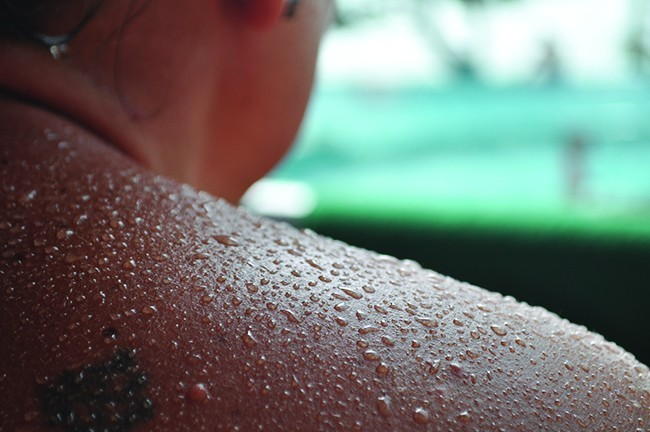Figure 4. Evaporation of sweat helps cool the body. (credit: “Kullez”/Flickr)

One way our body is cooled is by evaporation of the water in sweat (Figure 4). In very hot climates, we can lose as much as 1.5 L of sweat per day. Although sweat is not pure water, we can get an approximate value of the amount of heat removed by evaporation by assuming that it is. How much heat is required to evaporate 1.5 L of water (1.5 kg) at T = 37 °C (normal body temperature); ΔHvap = 43.46 kJ/mol at 37 °C.

How much heat is required to evaporate 100.0 g of liquid ammonia, NH3, at its boiling point if its enthalpy of vaporization is 4.8 kJ/mol?

## Melting and Freezing

When we heat a crystalline solid, we increase the average energy of its atoms, molecules, or ions and the solid gets hotter. At some point, the added energy becomes large enough to partially overcome the forces holding the molecules or ions of the solid in their fixed positions, and the solid begins the process of transitioning to the liquid state, or melting. At this point, the temperature of the solid stops rising, despite the continual input of heat, and it remains constant until all of the solid is melted. Only after all of the solid has melted will continued heating increase the temperature of the liquid (Figure 5).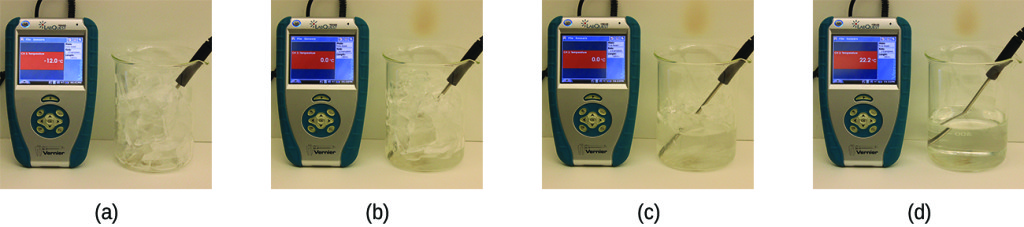Figure 5. (a) This beaker of ice has a temperature of −12.0 °C. (b) After 10 minutes the ice has absorbed enough heat from the air to warm to 0 °C. A small amount has melted. (c) Thirty minutes later, the ice has absorbed more heat, but its temperature is still 0 °C. The ice melts without changing its temperature. (d) Only after all the ice has melted does the heat absorbed cause the temperature to increase to 22.2 °C. (credit: modification of work by Mark Ott)

If we stop heating during melting and place the mixture of solid and liquid in a perfectly insulated container so no heat can enter or escape, the solid and liquid phases remain in equilibrium. This is almost the situation with a mixture of ice and water in a very good thermos bottle; almost no heat gets in or out, and the mixture of solid ice and liquid water remains for hours. In a mixture of solid and liquid at equilibrium, the reciprocal process of melting and freezing occur at equal rates, and the quantities of solid and liquid therefore remain constant. The temperature at which the solid and liquid phases of a given substance are in equilibrium is called the melting point of the solid or the freezing point of the liquid. Use of one term or the other is normally dictated by the direction of the phase transition being considered, for example, solid to liquid (melting) or liquid to solid (freezing).

The enthalpy of fusion and the melting point of a crystalline solid depend on the strength of the attractive forces between the units present in the crystal. Molecules with weak attractive forces form crystals with low melting points. Crystals consisting of particles with stronger attractive forces melt at higher temperatures.

The amount of heat required to change one mole of a substance from the solid state to the liquid state is the enthalpy of fusion, ΔHfus of the substance. The enthalpy of fusion of ice is 6.0 kJ/mol at 0 °C. Fusion (melting) is an endothermic process:

${\text{H}}_{2}\text{O}\left(s\right)\longrightarrow {\text{H}}_{2}\text{O(}l\text{)}\Delta {H}_{\text{fus}}=\text{6.01 kJ/mol}$

The reciprocal process, freezing, is an exothermic process whose enthalpy change is −6.0 kJ/mol at 0 °C:

${\text{H}}_{2}\text{O}\left(l\right)\longrightarrow {\text{H}}_{2}\text{O(}s\text{)}\Delta {H}_{\text{frz}}=-\Delta {H}_{\text{fus}}=-6.01\text{kJ/mol}$

## Sublimation and Deposition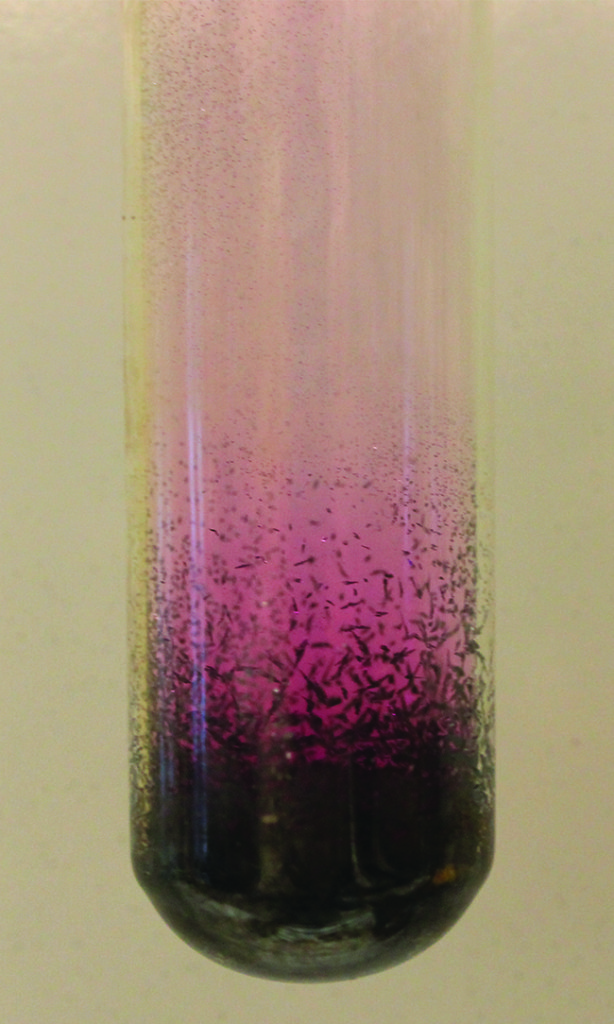Figure 6. Sublimation of solid iodine in the bottom of the tube produces a purple gas that subsequently deposits as solid iodine on the colder part of the tube above. (credit: modification of work by Mark Ott)

Some solids can transition directly into the gaseous state, bypassing the liquid state, via a process known as sublimation. At room temperature and standard pressure, a piece of dry ice (solid CO2) sublimes, appearing to gradually disappear without ever forming any liquid. Snow and ice sublime at temperatures below the melting point of water, a slow process that may be accelerated by winds and the reduced atmospheric pressures at high altitudes. When solid iodine is warmed, the solid sublimes and a vivid purple vapor forms (Figure 6). The reverse of sublimation is called deposition, a process in which gaseous substances condense directly into the solid state, bypassing the liquid state. The formation of frost is an example of deposition.

Like vaporization, the process of sublimation requires an input of energy to overcome intermolecular attractions. The enthalpy of sublimation, ΔHsub, is the energy required to convert one mole of a substance from the solid to the gaseous state. For example, the sublimation of carbon dioxide is represented by:

${\text{CO}}_{2}\left(s\right)\longrightarrow {\text{CO}}_{2}\text{(}g\text{)}\Delta {H}_{\text{sub}}=\text{26.1 kJ/mol}$

Likewise, the enthalpy change for the reverse process of deposition is equal in magnitude but opposite in sign to that for sublimation:

${\text{CO}}_{2}\left(g\right)\longrightarrow {\text{CO}}_{2}\text{(}s\text{)}\Delta {H}_{\text{dep}}=-\Delta {H}_{\text{sub}}=-26.1\text{kJ/mol}$

Consider the extent to which intermolecular attractions must be overcome to achieve a given phase transition. Converting a solid into a liquid requires that these attractions be only partially overcome; transition to the gaseous state requires that they be completely overcome. As a result, the enthalpy of fusion for a substance is less than its enthalpy of vaporization. This same logic can be used to derive an approximate relation between the enthalpies of all phase changes for a given substance. Though not an entirely accurate description, sublimation may be conveniently modeled as a sequential two-step process of melting followed by vaporization in order to apply Hess’s Law. Viewed in this manner, the enthalpy of sublimation for a substance may be estimated as the sum of its enthalpies of fusion and vaporization, as illustrated in Figure 7. For example:

$\begin{array}{l}\text{solid}\longrightarrow \text{liquid}\Delta {H}_{\text{fus}}\\ \underline{\text{liquid}\longrightarrow \text{gas}\Delta {H}_{\text{vap}}}\\ \text{solid}\longrightarrow \text{gas}\Delta {H}_{\text{sub}}=\Delta {H}_{\text{fus}}+\Delta {H}_{\text{vap}}\end{array}$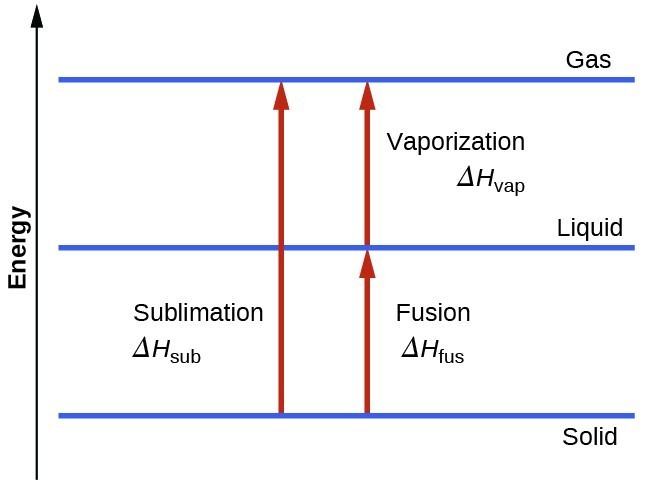Figure 7. For a given substance, the sum of its enthalpy of fusion and enthalpy of vaporization is approximately equal to its enthalpy of sublimation.

## Heating and Cooling Curves

In the chapter on thermochemistry, the relation between the amount of heat absorbed or related by a substance, q, and its accompanying temperature change, ΔT, was introduced:

$q=mc\Delta T$

where m is the mass of the substance and c is its specific heat. The relation applies to matter being heated or cooled, but not undergoing a change in state. When a substance being heated or cooled reaches a temperature corresponding to one of its phase transitions, further gain or loss of heat is a result of diminishing or enhancing intermolecular attractions, instead of increasing or decreasing molecular kinetic energies. While a substance is undergoing a change in state, its temperature remains constant. Figure 8 shows a typical heating curve.

Consider the example of heating a pot of water to boiling. A stove burner will supply heat at a roughly constant rate; initially, this heat serves to increase the water’s temperature. When the water reaches its boiling point, the temperature remains constant despite the continued input of heat from the stove burner. This same temperature is maintained by the water as long as it is boiling. If the burner setting is increased to provide heat at a greater rate, the water temperature does not rise, but instead the boiling becomes more vigorous (rapid). This behavior is observed for other phase transitions as well: For example, temperature remains constant while the change of state is in progress.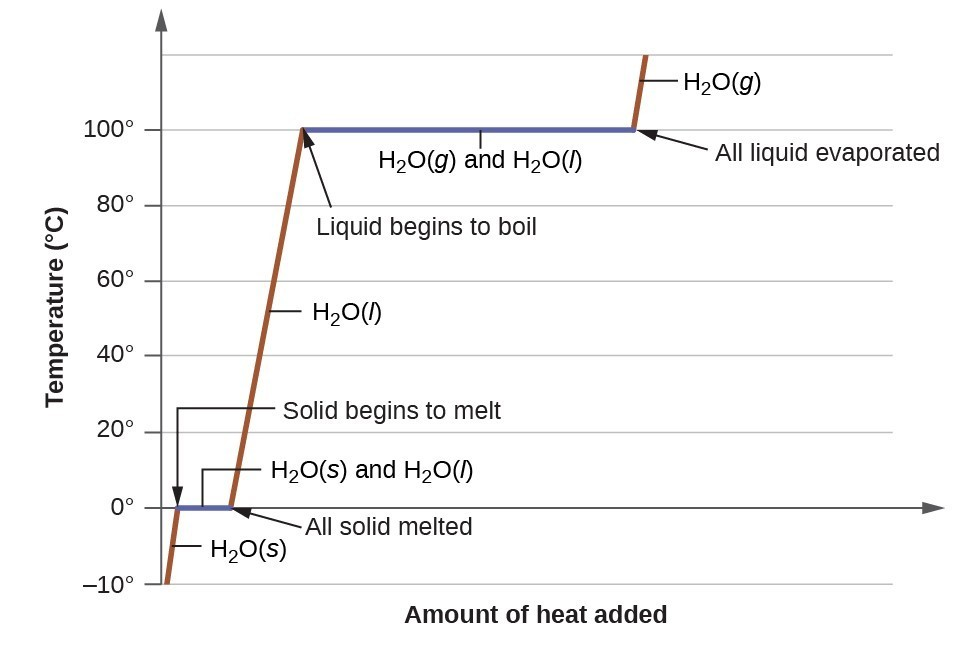Figure 8. A typical heating curve for a substance depicts changes in temperature that result as the substance absorbs increasing amounts of heat. Plateaus in the curve (regions of constant temperature) are exhibited when the substance undergoes phase transitions.

### Example 6: Total Heat Needed to Change Temperature and Phase for a Substance

How much heat is required to convert 135 g of ice at −15 °C into water vapor at 120 °C?

What is the total amount of heat released when 94.0 g water at 80.0 °C cools to form ice at −30.0 °C?

### Key Concepts and Summary

Phase transitions are processes that convert matter from one physical state into another. There are six phase transitions between the three phases of matter. Melting, vaporization, and sublimation are all endothermic processes, requiring an input of heat to overcome intermolecular attractions. The reciprocal transitions of freezing, condensation, and deposition are all exothermic processes, involving heat as intermolecular attractive forces are established or strengthened. The temperatures at which phase transitions occur are determined by the relative strengths of intermolecular attractions and are, therefore, dependent on the chemical identity of the substance.

#### Key Equations

• $P=A{e}^{-\Delta {H}_{\text{vap}}\text{/}RT}$
• $\text{ln}P=-\frac{\Delta {H}_{\text{vap}}}{RT}+\text{ln}A$
• $\text{ln}\left(\frac{{P}_{2}}{{P}_{1}}\right)=\frac{\Delta {H}_{\text{vap}}}{R}\left(\frac{1}{{T}_{1}}-\frac{1}{{T}_{2}}\right)$

### Exercises

1. Heat is added to boiling water. Explain why the temperature of the boiling water does not change. What does change?
2. Heat is added to ice at 0 °C. Explain why the temperature of the ice does not change. What does change?
3. What feature characterizes the dynamic equilibrium between a liquid and its vapor in a closed container?
4. Identify two common observations indicating some liquids have sufficient vapor pressures to noticeably evaporate?
5. Identify two common observations indicating some solids, such as dry ice and mothballs, have vapor pressures sufficient to sublime?
6. What is the relationship between the intermolecular forces in a liquid and its vapor pressure?
7. What is the relationship between the intermolecular forces in a solid and its melting temperature?
8. Why does spilled gasoline evaporate more rapidly on a hot day than on a cold day?
9. Carbon tetrachloride, CCl4, was once used as a dry cleaning solvent, but is no longer used because it is carcinogenic. At 57.8 °C, the vapor pressure of CCl4 is 54.0 kPa, and its enthalpy of vaporization is 33.05 kJ/mol. Use this information to estimate the normal boiling point for CCl4.
10. When is the boiling point of a liquid equal to its normal boiling point?
11. How does the boiling of a liquid differ from its evaporation?
12. Use the information in Figure 3 to estimate the boiling point of water in Denver when the atmospheric pressure is 83.3 kPa.
13. A syringe at a temperature of 20 °C is filled with liquid ether in such a way that there is no space for any vapor. If the temperature is kept constant and the plunger is withdrawn to create a volume that can be occupied by vapor, what would be the approximate pressure of the vapor produced?
14. Explain the following observations:
1. It takes longer to cook an egg in Ft. Davis, Texas (altitude, 5000 feet above sea level) than it does in Boston (at sea level).
2. Perspiring is a mechanism for cooling the body.
15. The enthalpy of vaporization of water is larger than its enthalpy of fusion. Explain why.
16. Explain why the molar enthalpies of vaporization of the following substances increase in the order CH4 < C2H6 < C3H8, even though all three substances experience the same dispersion forces when in the liquid state.
17. Explain why the enthalpies of vaporization of the following substances increase in the order CH4 < NH3 < H2O, even though all three substances have approximately the same molar mass.
18. The enthalpy of vaporization of CO2(l) is 9.8 kJ/mol. Would you expect the enthalpy of vaporization of CS2(l) to be 28 kJ/mol, 9.8 kJ/mol, or −8.4 kJ/mol? Discuss the plausibility of each of these answers.
19. The hydrogen fluoride molecule, HF, is more polar than a water molecule, H2O (for example, has a greater dipole moment), yet the molar enthalpy of vaporization for liquid hydrogen fluoride is lesser than that for water. Explain.
20. Ethyl chloride (boiling point, 13 °C) is used as a local anesthetic. When the liquid is sprayed on the skin, it cools the skin enough to freeze and numb it. Explain the cooling effect of liquid ethyl chloride.
21. Which contains the compounds listed correctly in order of increasing boiling points?
1. N2 < CS2 < H2O < KCl
2. H2O < N2 < CS2 < KCl
3. N2 < KCl < CS2 < H2O
4. CS2 < N2 < KCl < H2O
5. KCl < H2O < CS2 < N2
22. How much heat is required to convert 422 g of liquid H2O at 23.5 °C into steam at 150 °C?
23. Evaporation of sweat requires energy and thus take excess heat away from the body. Some of the water that you drink may eventually be converted into sweat and evaporate. If you drink a 20-ounce bottle of water that had been in the refrigerator at 3.8 °C, how much heat is needed to convert all of that water into sweat and then to vapor?? (Note: Your body temperature is 36.6 °C. For the purpose of solving this problem, assume that the thermal properties of sweat are the same as for water.)
24. Titanium tetrachloride, TiCl4, has a melting point of −23.2 °C and has a ΔH fusion = 9.37 kJ/mol.
1. How much energy is required to melt 263.1 g TiCl4?
2. For TiCl4, which will likely have the larger magnitude: ΔH fusion or ΔH vaporization? Explain your reasoning.

## Glossary

boiling point: temperature at which the vapor pressure of a liquid equals the pressure of the gas above it

Clausius-Clapeyron equation: mathematical relationship between the temperature, vapor pressure, and enthalpy of vaporization for a substance

condensation: change from a gaseous to a liquid state

deposition: change from a gaseous state directly to a solid state

dynamic equilibrium: state of a system in which reciprocal processes are occurring at equal rates

freezing: change from a liquid state to a solid state

freezing point: temperature at which the solid and liquid phases of a substance are in equilibrium; see also melting point

melting: change from a solid state to a liquid state

melting point: temperature at which the solid and liquid phases of a substance are in equilibrium; see also freezing point

normal boiling point: temperature at which a liquid’s vapor pressure equals 1 atm (760 torr)

sublimation: change from solid state directly to gaseous state

vapor pressure: (also, equilibrium vapor pressure) pressure exerted by a vapor in equilibrium with a solid or a liquid at a given temperature

vaporization: change from liquid state to gaseous state